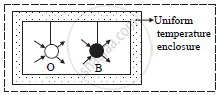# Prove Kirchhoff’S Law of Radiation Theoretically. - Physics

Prove Kirchhoff’s law of radiation theoretically.

#### Solution

Consider an ordinary body O and perfectly black body B of the same dimension suspended in a uniform temperature enclosure as shown in the figure.
At thermal equilibrium, both the bodies will have the same temperature as that of the enclosure.
Let, E = emissive power of ordinary body O
Eb = emissive power of perfectly black body B
a = coefficient of absorption of O
e = emissivity of O
Q = radiant energy incident per unit time per unit area on each bodyQuantity of heat absorbed per unit area per unit time by body O = aQ.
Quantity of heat energy emitted per unit area per unit time by body O = E.
Since there is no change in temperature
E = aQ

Q = E/a  .…(1)

Quantity of heat absorbed per unit area per unit time by a perfectly black body, B = Q The radiant heat energy emitted per unit time per unit area by a perfectly black body,
B = Eb
Since there is no change in temperature.
Eb = Q   .…(2)

From equations (1) and (2),

E/a=E_b =>E/E_b=a

But, E/E_b=e

a = e

Concept: Kirchhoff’s Law of Heat Radiation and Its Theoretical Proof
Is there an error in this question or solution?

#### APPEARS IN

Balbharati Physics 12th Standard HSC Maharashtra State Board
Chapter 3 Kinetic Theory of Gases and Radiation
Exercises | Q 10 | Page 73